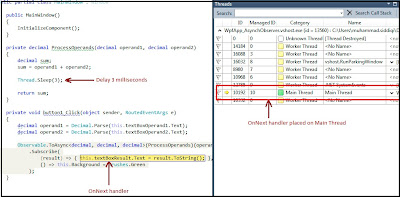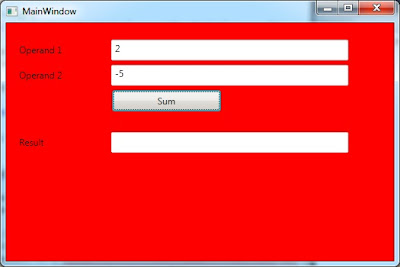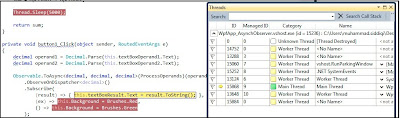## Tuesday, February 22, 2011

### WPF - ASynchronous Function using Observable.ToASync [Rx ASynchronous Delegate] - Part # 1

This is the first part of our discussion about asynchronous method execution using Reactive Extension. The second part of this discussion can be found here:

http://shujaatsiddiqi.blogspot.com/2011/02/wpf-asynchronous-function-using_23.html

In this post we discuss how we can execute a method asynchronously using the features provided in Reactive Extensions Rx. We discussed the usage of asynchronous delegate in a WPF application in the following post:

http://shujaatsiddiqi.blogspot.com/2010/12/asynchronous-delegate-exception-
model.html

This is basically the similar concept in Rx.
`<Window x:Class="WpfApp_AsynchObserver.MainWindow"        xmlns="http://schemas.microsoft.com/winfx/2006/xaml/presentation"        xmlns:x="http://schemas.microsoft.com/winfx/2006/xaml"        Title="MainWindow" Height="350" Width="525">    <Grid>        <TextBox Height="27" HorizontalAlignment="Left" Margin="138,22,0,0"                       Name="textBoxOperand1" VerticalAlignment="Top" Width="311" />        <TextBox Height="27" HorizontalAlignment="Left" Margin="138,55,0,0"                       Name="textBoxOperand2" VerticalAlignment="Top" Width="311" />        <TextBox Height="27" HorizontalAlignment="Left" Margin="138,143,0,0"                      Name="textBoxResult" VerticalAlignment="Top" Width="311" />        <Button Content="Sum" Height="28" HorizontalAlignment="Left" Margin="139,88,0,0"                              Name="button1" VerticalAlignment="Top" Width="143" Click="button1_Click" />        <Label Content="Operand 1" Height="27" HorizontalAlignment="Left" Margin="12,22,0,0"                            Name="label1" VerticalAlignment="Top" Width="120" />        <Label Content="Operand 2" Height="27" HorizontalAlignment="Left" Margin="12,55,0,0"                        Name="label2" VerticalAlignment="Top" Width="120" />        <Label Content="Result" Height="27" HorizontalAlignment="Left" Margin="12,143,0,0"                         Name="label3" VerticalAlignment="Top" Width="120" />    </Grid></Window>`The code behind is as follows:
`public partial class MainWindow : Window{    public MainWindow()    {        InitializeComponent();    }    private decimal ProcessOperands(decimal operand1, decimal operand2)    {        decimal sum;        sum = operand1 + operand2;        return sum;    }    private void button1_Click(object sender, RoutedEventArgs e)    {        decimal operand1 = Decimal.Parse(this.textBoxOperand1.Text);        decimal operand2 = Decimal.Parse(this.textBoxOperand2.Text);        this.textBoxResult.Text = ProcessOperands(operand1, operand2).ToString();    }}`

Lets run the application now. Everything is working great. We enter numeric digits in the two operands fields. When we click Enter, the result appears in the Result text box. Now let us change the method call (ProcessOperands) to be an asynchronous call using Observable.ToAsync. We need to update the button’s click handler as follows:
`private void button1_Click(object sender, RoutedEventArgs e){    decimal operand1 = Decimal.Parse(this.textBoxOperand1.Text);    decimal operand2 = Decimal.Parse(this.textBoxOperand2.Text);    Observable.ToAsync<decimal, decimal, decimal>(ProcessOperands)(operand1, operand2)        .Subscribe(                     (result) => { this.textBoxResult.Text = result.ToString(); }                  );}`

The code clearly shows that we have used an overload of ToAsync which takes two decimal values as arguments and returns a decimal value. We have used this as our ProcessOperand method is defined like that. You can see that we have used the value provided in the onNext parameter to populate the textBoxResult. Basically onNext is placed when the async code is finished execution and result is available. If we compare it to the code we have written for Asynchronous delegates, we realize that Rx has internally executed code for BeginInvoke and also called EndInvoke for us getting the result using IAsyncResult. If we look at the marble diagram, it should be like this.In the marble diagram, this has shown to be placing an OnCompleted message afterwards. Let us verify that this event is generated. In the following code, we are updating the Background color to green when OnCompleted message is received from the Observable generated for the method.
`private void button1_Click(object sender, RoutedEventArgs e){    decimal operand1 = Decimal.Parse(this.textBoxOperand1.Text);    decimal operand2 = Decimal.Parse(this.textBoxOperand2.Text);    Observable.ToAsync<decimal, decimal, decimal>(ProcessOperands)(operand1, operand2)        .Subscribe(                     (result) => { this.textBoxResult.Text = result.ToString(); },                     () => this.Background = Brushes.Green                  );}`

Now we run the application and enter values in the operands text boxes. When we click the button, the textBoxResult is updated with the sum of two operands. The window also turns green. This is due to the code we have written in OnCompleted handler.Long running methods and Rx Thread Management for generating asynchronous block:
Now you might be thinking that this is a very simple example. What if the method is a long running method taking a few seconds. Would it still be the same code. Let’s mimic that this method is taking a few seconds by putting Thread.Sleep in method code as follows:
`private decimal ProcessOperands(decimal operand1, decimal operand2){    decimal sum;    sum = operand1 + operand2;    Thread.Sleep(5000);}`

If you run this and enter data in the input text boxes and click Sum. You get the following exception:Why is this exception generated by just delaying in the asynchronous method? Basically we have caused the subscription to be on a ThreadPool thread. Since we are delaying for 5 seconds, all Rx messages (OnNext, OnCompleted and OnError) seem to be dispatched on the calling thread of subscriber.

It seems if there is a delay of more than 3 milliseconds then the OnNext messages are dispatched on a ThreadPool thread otherwise they are dispatched on the thread of the subscriber. In this case it is UI thread. If the delay is 3 milliseconds or lesser these messages are always dispatched on UI thread. This is true even though we know that ToAsync is causing the method to be executed on a ThreadPool thread.

Fig: When OnNext is received in more than 3 milliseconds:Fig: When OnNext is received in 3 milliseconds or less:How exceptions are handled?

As we have discussed [http://shujaatsiddiqi.blogspot.com/2010/12/asynchronous-delegate-exception-model.html] the runtime is silent about exceptions for Async delegates if we don’t call EndInvoke. It is not the case with Observable.ToAsync. It always generate exception message OnError when there is an exception in the method executed asynchronously. Let us change the code of ProcessOperands so that it throws an exception.
`[DebuggerStepThrough]private decimal ProcessOperands(decimal operand1, decimal operand2){    decimal sum;    sum = operand1 + operand2;    if (sum < 0)    {        throw new System.Exception("Exception in processing data!");    }    return sum;}`

The above code is resulting an exception if the sum of these two operands is negative. We also need to update the code so that IObservable generated from Observable.ToAsync has a non-default OnError handler. I have decorated the method with DebuggerStepThrough attribute so that Debugger doesn’t bother me as I have FCEs turned on. Let’s update button1_Click as follows:
`private void button1_Click(object sender, RoutedEventArgs e){    decimal operand1 = Decimal.Parse(this.textBoxOperand1.Text);    decimal operand2 = Decimal.Parse(this.textBoxOperand2.Text);    Observable.ToAsync<decimal, decimal, decimal>(ProcessOperands)(operand1, operand2)        .Subscribe(            (result) => { this.textBoxResult.Text = result.ToString(); },            (ex) => this.Background = Brushes.Red,            () => this.Background = Brushes.Green        );}`

It just changes the background of the window to Red if there is an OnError message from the method. Let’s run the application and enter data 2 and -5 in the operand fields. As we know that their sum is -3, the background of the window should turn Red.We can show this in marble diagram as follows:Observing on Non-UI thread using different IScheduler:

Now we suppose that the method would always take more than 3 milliseconds. Can we still use ToAsync feature of Observable? Yes we can!

We just need to Observe on the Dispatcher. This can be done by using ObserveOn feature of IObservable. There are two methods provided for this purpose. One method allows us to generate the messages on any IScheduler (including built-in schedulers like Dispatcher, ThreadPool, TaskPool, Immediate, CurrentThread, NewThread). We can use any of the four overloads of this method. We can also directly use ObserveOnDispatcher method from IObservable. We have used the same as below.
`private void button1_Click(object sender, RoutedEventArgs e){    decimal operand1 = Decimal.Parse(this.textBoxOperand1.Text);    decimal operand2 = Decimal.Parse(this.textBoxOperand2.Text);    Observable.ToAsync<decimal, decimal, decimal>(ProcessOperands)(operand1, operand2)        .ObserveOnDispatcher<decimal>()        .Subscribe(            (result) => { this.textBoxResult.Text = result.ToString(); },            (ex) => this.Background = Brushes.Red,            () => this.Background = Brushes.Green        );}`

Now we run the application. Although the method is being executed on a separate ThreadPool thread but the OnNext, OnError and OnCompleted messages are dispatched on UI thread.Fig: OnNext message generated on Main UI ThreadFig: OnCompleted placed on Main UI threadFig: OnError placed on Main UI threadLimitations:
Since this is a way to implement Anonymous asynchronous delegates using Reactive Extensions so it seems to have has the same limitation as anonymous asynchronous delegate (Func) has. We can not have out or ref parameters in the method that we want to execute asynchronously. In non-reactive model, we might easily implement named asynchronous delegate as a work around but in the reactive extension world there doesn't seem to be any alternative.